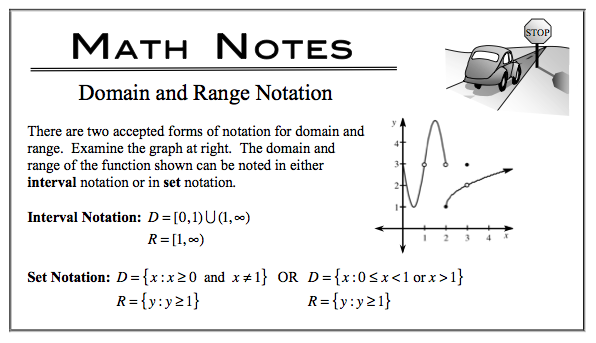### Home > APCALC > Chapter 1 > Lesson 1.2.6 > Problem1-94

1-94.

If $f(x) = (x - 3)^2 + 4$ and $g(x) =\frac { 1 } { x - 4 }-2$ , write explicit equations for the following transformations. Homework Help ✎

1. $h(x) = f(x + 2) - 5$

h(x) = f(x + 2) = 5
h(x) = [((x + 2) − 3) 2] + 4 − 5
Simplify.

2. $k(x) = g(3 - x) + 2$

$(3 − x)$, the horizontal shift, will replace '$x$' in the $g(x)$ function.
$+2$, the vertical shift, will be added to the entire '$g(x)$' function.

3. Use set notation to state the domains and ranges of $h$ and $k$.

Consider the parent graphs of $f$ and $g$.
Domain and range will shift from that of the parent.
The parent graph of $f(x)$ is $y = x^2$.
What is the parent graph of $g(x)$?